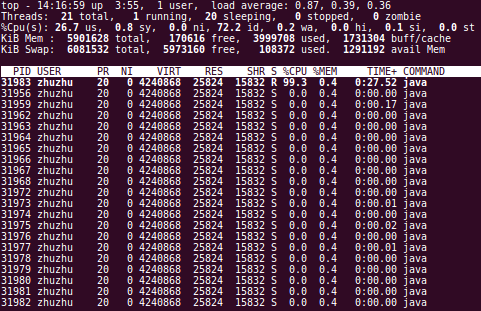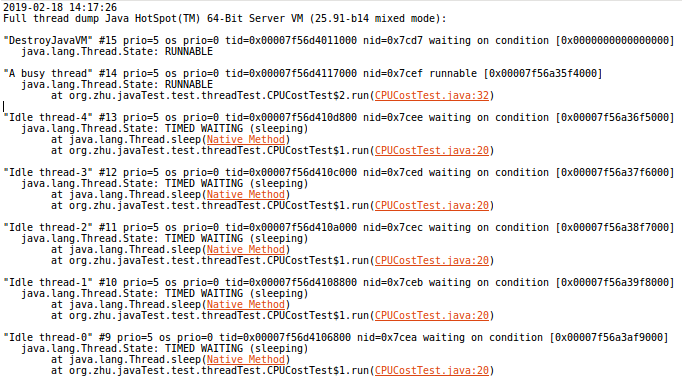It is common that using threads to deal with tasks simultaneously in Java programs. So it is important and useful to find which thread in JVM that costs CPU most.

## Using Top and jstack Command

One of the methods is using top and jstack command.

Here is an example that starting multiple threads:

``````public class CPUCostTest {
public static void main(String... strings) {
for (int i = 0; i < num_thread; i++) {
public void run() {
long interval_time = 100 * 1000;
try {
} catch (Exception e) {
}
}
};
t.start();
}
public void run() {
int i = 1;
while (true) {
i = (i++) / 5;
}
}
};
t.start();
}
}
``````

In this program, it starts 10 idle threads doing nothing but waiting and 1 busy thread that keeps calculating.

Run this program and find its PID.

Use `top -Hp PID` to check all the threads in this process. We will get:All the threads in this process are list with PID, CPU, Memory, etc. Then we could find the thread that costs CPU most(the first thread in the image above).

After this, we can use `jstack PID` to find the stack by the nid which in fact is the hexadecimal value of PID.In this image, the thread named “A busy thread” is what we are looking for.